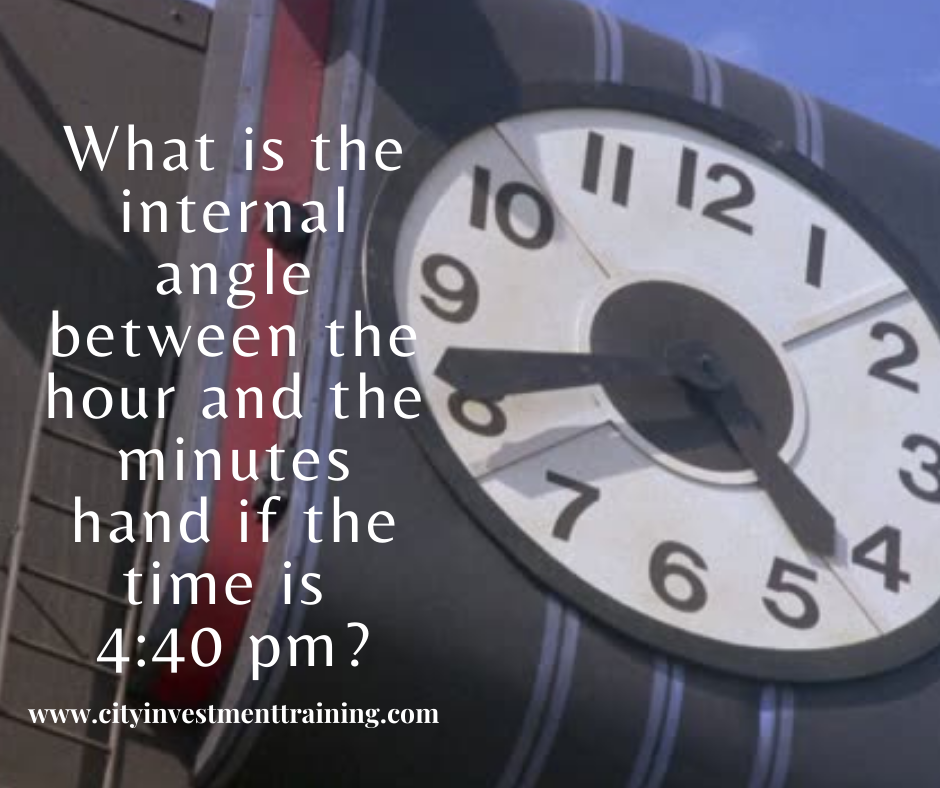Search

# What is the internal angle between the hour and the minutes hand if the time is 4:40 pm?

Check our popular ebook "Top 100 Investment Banking Questions with Answers".### What is the internal angle between the hour and the minutes hand if the time is 4:40 pm?

To tee off, what will be handy is to first compute the angles for everyone hour or five minutes. From there on we can work out the angles for any time.

The number of degrees in one hour

We know that a circle consists of 360 degrees.

The clock has 12 hours, so we need to divide 360 by 12 to get the degree for every hour or five minutes.

i.e. 360/12 we get 30 degrees in every one hour or five minutes

The angle between 4 hour and 40 minutes

The minutes hand at 40 minutes means the same as the hour hand at 8 pm.

So, for working out the angle for 4:40 we get

8 – 4 =4 hours

The angle for four hours is 4*30 degrees = 120 degrees

Be careful – it ain’t over yet – this is what separates a good candidate from a great one.

The angle at 4:40 pm

Remember at time 4:40, the hour hand does not stand still at four but has started to make its way toward five. The question is how much?

If the time is 4:40 then the minutes hand has moved 2/3rd its way towards 12 and so the hours hand would have moved by the same proportion i.e. 2/3rd which gives us an angle of 2/3rd of an hour or 2/3rd of 30 degrees i.e. 20 degrees.

We will now subtract this from 120 degrees to get 100 degrees.